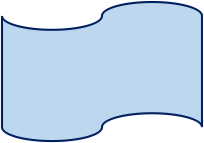Study Guide

# Basic Geometry - Polygons

## Polygons

A polygon is any closed figure with three or more straight sides. "Closed" means that there are no gaping holes in it and that all sides connect together.

### These Are Polygons:### These Are Not Polygons:The first shape is a line, which is definitely not a shape with more than three sides. Sorry brah, you're out. The next shape has more than three sides, but they don't all connect. Bad news dude, you're out too. That's right, a circle is not a polygon, either. While it is a closed figure, it's really hard to count the number of sides a circle has because it has none. The final shape is closed, and it does have more than three sides, but two of the sides are curved. They aren't straight lines, so again, sorry Charlie.

### Names of Some Common Polygons

 Number of Sides Number of Angles Name 3 3 Triangle 4 4 Quadrilateral 5 5 Pentagon 6 6 Hexagon 7 7 Septagon (or Heptagon) 8 8 Octagon 9 9 Nonagon 10 10 Decagon 12 12 Dodecagon

### Important Vocabulary

Polygons have a lot of important vocabulary terms that come with them. You will need to memorize these, but don't worry, they aren't too difficult.

• Angle: the shape formed when two rays meet at a common point. AKA "the corner."
• Vertex: the point where two rays meet; the corner point of a polygon. The plural of vertex is vertices.
• Side: the straight edge of a polygon.• Convex Polygon: a polygon with no interior angle greater than 180°.
• Concave Polygon: a polygon with at least one interior angle greater than 180°.
• Equiangular: a figure where all angles are equal in measure.
• Equilateral: a figure where all sides are equal in length.
• Regular Polygon: an equilateral, equiangular polygon.

Polygons can be convex or concave. Convex polygons are the ones we're used to seeing the most: squares, triangles, pentagons, etc. All vertices in convex polygons point outward away from the center. Officially, each interior angle in a convex polygon is less than 180° , and this is what makes all of the vertices point out. These are examples of convex polygons.If we draw a line through any of these convex polygons, the line will cross through only 2 sides of the polygon.Concave polygons have some angles that point in toward the center, like a cave, for con-men… a concave… get it? An example of a concave polygon is a 5-pointed star. Notice that some of the angles point away from the center, like the points of the star, and some point in toward the center like the angles between the star's points. A concave polygon has one or more interior angles that are greater than 180°. Here are some examples of concave polygons.If we draw a line through any of these concave polygons, it's possible that the line could cross through more than just two sides.### Equiangular and Equilateral

Some polygons like to play fair. They make sure all their angles are the exact same measure so no one angle is bigger or smaller than any other angle. These are called equiangular polygons. Rectangles are equiangular because all four angles are 90°. The sides don't have to be equal lengths for the polygon to be equiangular.Some polygons like to have all their sides the same length, they are called equilateral. A rhombus is an example of an equilateral polygon because it has four equal sides, even though the angles aren't equal.And then there are those polygons that are really nice and want all of their side lengths equal and all of their angle measures equal. That's one super nice, equiangular and equilateral polygon. It's so nice it gets its own name: it's a regular polygon.

### Regular Polygons

You're probably familiar with these shapes. All of their sides are the same length and all of their angles are the same measure.### These Are Not Regular### Base and Height of a Polygon

• Base: the bottom side of a polygon.
• Height: the height of a polygon is the perpendicular distance from the top-most vertex to the base. The height and base ALWAYS form a right angle.